# Laplace Transform

Laplace transform converts a time domain function to s-domain function by integration from zero to infinity

of the time domain function, multiplied by e-st.

The Laplace transform is used to quickly find solutions for differential equations and integrals.

Derivation in the time domain is transformed to multiplication by s in the s-domain.

Integration in the time domain is transformed to division by s in the s-domain.

## Laplace transform function

The Laplace transform is defined with the L{} operator: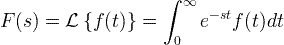### Inverse Laplace transform

The inverse Laplace transform can be calculated directly.

Usually the inverse transform is given from the transforms table.

## Laplace transform table

Function name Time domain function Laplace transform

f (t)

F(s) = L{f (t)}

Constant 1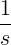Linear t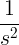Power

t n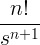Power

t a

Γ(a+1) ⋅ s -(a+1)

Exponent

e at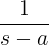Sine

sin at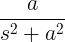Cosine

cos at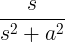Hyperbolic sine

sinh at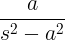Hyperbolic cosine

cosh at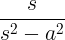Growing sine

t sin at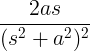Growing cosine

t cos at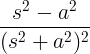Decaying  sine

e -at sin ωt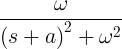Decaying cosine

e -at cos ωt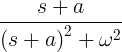Delta function

δ(t)

1

Delayed delta

δ(t-a)

e-as

## Laplace transform properties

Property name Time domain function Laplace transform Comment

f (t)

F(s)

Linearity a f (t)+bg(t) aF(s) + bG(s) a,b are constant
Scale change f (at)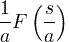a>0
Shift e-at f (t) F(s + a)
Delay f (t-a) e-asF(s)
Derivation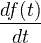sF(s) - f (0)
N-th derivation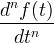snf (s) - sn-1f (0) - sn-2f '(0)-...-f (n-1)(0)
Power t n f (t)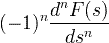Integration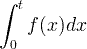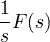Reciprocal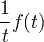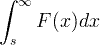Convolution f (t) * g (t) F(s) ⋅ G(s) * is the convolution operator
Periodic function f (t) = f (t+T)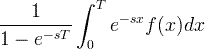## Laplace transform examples

#### Example #1

Find the transform of f(t):

f (t) = 3t + 2t2

Solution:

ℒ{t} = 1/s2

ℒ{t2} = 2/s3

F(s) = ℒ{f (t)} = ℒ{3t + 2t2} = 3ℒ{t} + 2ℒ{t2} = 3/s2 + 4/s3

#### Example #2

Find the inverse transform of F(s):

F(s) = 3 / (s2 + s - 6)

Solution:

In order to find the inverse transform, we need to change the s domain function to a simpler form:

F(s) = 3 / (s2 + s - 6) = 3 / [(s-2)(s+3)] = a / (s-2) + b / (s+3)

[a(s+3) + b(s-2)] / [(s-2)(s+3)] = 3 / [(s-2)(s+3)]

a(s+3) + b(s-2) = 3

To find a and b, we get 2 equations - one of the s coefficients and second of the rest:

(a+b)s + 3a-2b = 3

a+b = 0 , 3a-2b = 3

a = 3/5 , b = -3/5

F(s) = 3 / 5(s-2) - 3 / 5(s+3)

Now F(s) can be transformed easily by using the transforms table for exponent function:

f (t) = (3/5)e2t - (3/5)e-3t